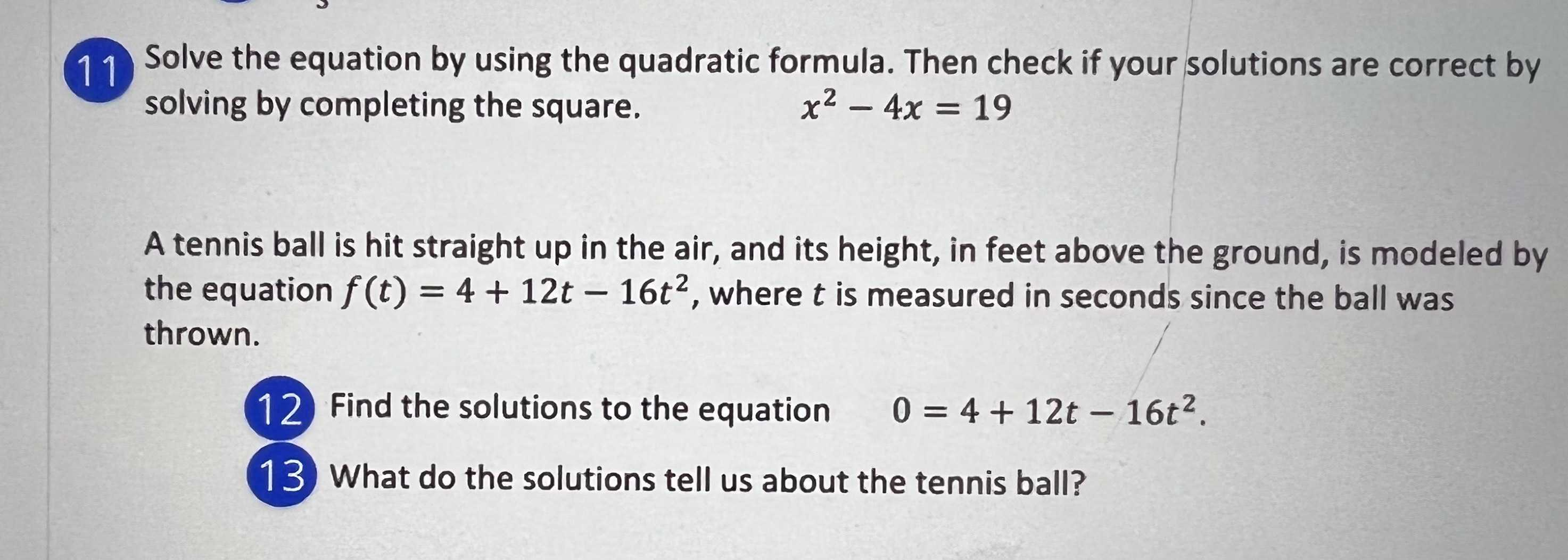### ¿Todavía tienes preguntas de matemáticas?

Pregunte a nuestros tutores expertos
Algebra
Pregunta11.Solve the equation by using the quadratic formula. Then check if your solutions are correct by solving by completing the square. $$x ^ { 2 } - 4 x = 19$$

A tennis ball is hit straight up in the air, and its height, in feet above the ground, is modeled by the equation $$f ( t ) = 4 + 12 t - 16 t ^ { 2 }$$ , where $$t$$ is measured in seconds since the ball was thrown.

12. Find the solutions to the equation $$0 = 4 + 12 t - 16 t ^ { 2 }$$ .

13. What do the solutions tell us about the tennis ball?